# Isosceles triangle

The circumference of the isosceles triangle is 32.5 dm. Base length is 153 cm. How long is the leg of this triangle?

Result

r =  8.6 dm

#### Solution:

$o=32.5 \ \text{dm} \ \\ a=153 \ cm=153 / 10 \ dm=15.3 \ dm \ \\ \ \\ o=a + 2r \ \\ r=(o-a)/2=(32.5-15.3)/2=\dfrac{ 43 }{ 5 }=8.6 \ \text{dm}$

Try calculation via our triangle calculator.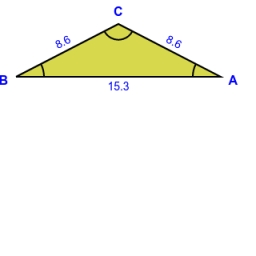Our examples were largely sent or created by pupils and students themselves. Therefore, we would be pleased if you could send us any errors you found, spelling mistakes, or rephasing the example. Thank you!

Leave us a comment of this math problem and its solution (i.e. if it is still somewhat unclear...):Be the first to comment!Tips to related online calculators

## Next similar math problems:

1. Arm-legCalculate the length of the base of an isosceles triangle with a circumference 224 cm if the arm length is 68 cm.
2. TrianglesEquilateral triangle with side 40 cm has the same perimeter as an isosceles triangle with arm of 45 cm. Calculate the base x of an isosceles triangle.
3. Sides of triangle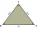Triangle circumference with two identical sides is 117cm. The third side measures 44cm. How many cms do you measure one of the same sides?
4. PerimetersA rectangle has a perimeter of 16p centimeters, it had a width of 2p centimeters. Each side of an equilateral triangle is 1/2 the length of the rectangle. Find the total perimeter of the rectangle and the triangle if p=8.
5. 7 triangle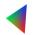The triangle area is 26.7 cm2. Determine the side length l if appropriate height hl = 45.3 cm.
6. An equilateral triangle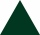The perimeter of an equilateral triangle is 33cm. How long is each side?
7. Two anglesThe triangles ABC and A'B'C 'are similar. In the ABC triangle, the two angles are 25° and 65°. Explain why in the triangle A'B'C 'is the sum of two angles of 90 degrees.
8. AnglesThe triangle is one outer angle 158°54' and one internal angle 148°. Calculate the other internal angles of a triangle.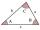The size of two internal angles of a triangle ABC are α=6/18π and β=7/18π. Calculate the size of the third angle.
10. 3-bracketMay be the largest angle in the triangle less than 20°?
11. Angles in triangle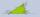Calculate the alpha angle in the triangle if beta is 61 degrees and 98 gamma degrees.
12. Bicycle wheelAfter driving 157 m bicycle wheel rotates 100 times. What is the radius of the wheel in cm?
13. Parallelogram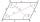In the parallelogram we know one internal angle 67°33`. Calculate the other internal angles.
14. HotelThe hotel has a p floors each floor has i rooms from which the third are single and the others are double. Represents the number of beds in hotel.
15. SimplifySimplify the following problem and express as a decimal: 5.68-[5-(2.69+5.65-3.89) /0.5]
16. ExpressionSolve for a specified variable: P=a+4b+3c, for a
17. Angles 1It is true neighboring angles have not common arm?